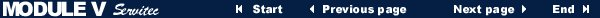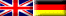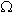MODULE V Electrical engineering - Basics Page 172.4 Exercises

1. A copper cable is 20m long and has a cross section of 4mm2. Determine the conductor resistance at a temperature of 20°C.

2. The conductor resistance of an aluminium cable that has a cross section of 2,5mm2 should maximally be 2,8at a temperature of 20°C. Calculate the maximum cable length in millimetres.

3. Determine the resistivity of an electric resistor that is 2cm long and has a cross section of 4mm2 and a resistance of 10.

4. The voltage in an electric circuit is 50V. Two resistors are connected in parallel:
R1 = 5and R2 = 20. Calculate:
a) The partial currents I1 and I2
b) The total amperage I
c) The substitute resistance R

5. In an electric storage heater three equal heating resistors (5kW each) have been installed.
Determine:
a) The amperages I1, I2, I3 and I
b) The resistance R1, R2 and R3
c) The total substitute resistance R• • Copyright © IWB e.V. 1996-2000 • Design, technical realisation and webmaster: ohlendorf@ohlendorf.de • •
• • Sponsored by EDUVINET • 800 x 600 recommended • •